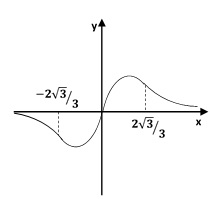# Let f(x) = (12x)/(9x^{2} + 4). Note that f(x) has odd symmetry: f(-x) = 12(-x)/(9(-x)^{2} + 4) =...

## Question:

Let {eq}f(x) = \frac{12x}{9x^{2} + 4} {/eq}. Note that {eq}f(x) {/eq} has odd symmetry:

$$f(-x) = \frac{12(-x)}{9(-x)^{2} + 4} = -\frac{12x}{9x^{2} + 4} = - f(x)$$

a) Determine the asymptotes and the signs of {eq}f(x) {/eq}. You can be brief in explaining your asymptotes.

b) Determine where {eq}f(x) {/eq} is increasing and where it is decreasing.

c) Determine where {eq}f(x) {/eq} is concave upwards and where it is concave downwards. What are the inflection points of {eq}f(x) {/eq}?

d) Produce a graph of {eq}y = f(x) {/eq}, labeling the information you learned in parts a) through c). Incorporate the symmetry mentioned at the beginning.

## Concavity and inflection point :

An inflection point is characterized by the fact that the function changes its concavity (it goes from concave to convex, or from convex to concave):

- A function is concave (concave up) at a point when the function falls above the tangent at that point.

- A function is convex (concave down) at a point when the function falls below the tangent at that point.

Parts a. Determine the asymptotes and the signs:

Domain . {eq}\displaystyle f(x) = \frac{12x}{(9x^2+4)}\\ \boxed{\displaystyle D = \{ x \in R \}}\\ \displaystyle x=0 \,\,\, \rightarrow \,\,\, y= 0\\ \displaystyle \boxed{ \left (0; 0 \right ) } \Longrightarrow \textrm {interception point with the y-axis and the x-axis}\\ {/eq}

Find vertical and horizontal asymptotes if any

Vertical Asymptotes {eq}\displaystyle \Longrightarrow \textrm {The functions has no vertical asymptote} {/eq}

Horizontal and Oblique asymptotes {eq}\displaystyle m= \lim_{x \rightarrow \infty } \frac{f(x)}{x} \\ \displaystyle m= \lim_{x \rightarrow \infty } \frac{12x}{x(9x^2+4)} \Longrightarrow \textrm {Applying l'Hospital's rule} \\ \displaystyle m= 0\\ \displaystyle b= \lim_{x \rightarrow \infty } (f(x) -mx\\ \displaystyle b= \lim_{x \rightarrow \infty } \frac{12x}{(9x^2+4)}\\ \displaystyle b= 0 \Longrightarrow \textrm {Applying l'Hospital's rule} {/eq}

Conclusion (Horizontal and Oblique asymptotes)

{eq}\displaystyle \boxed{ y= 0} \Longrightarrow \textrm {horizontal asymptote} {/eq}

To define the sign of the function in each interval, evaluate a point of each interval and verify the sign:

{eq}\left (-\infty, 0 \right) \,\,\,\, \rightarrow \,\,\,\, f < 0 \,\,\,\,\, \textrm { the function is negative in this interval }\\ \left (0,\infty\right) \,\,\,\, \rightarrow \,\,\,\, f > 0 \,\,\,\,\, \textrm { the function is positive in this interval }\\ {/eq}

Parts b. Monotony:

Find Critical points and intervals of increase/decrease

To find the intervals where the function is increasing and decreasing, we need to find and analyze the first derivative by finding all critical points:

{eq}\displaystyle f(x) = \frac{12x}{9x^2+4}\\ \displaystyle f'(x) =12\cdot \frac{1\cdot \left(9x^2+4\right)-18x^2}{\left(9x^2+4\right)^2}\\ \displaystyle f'(x) = \frac{12\left(-9x^2+4\right)}{\left(9x^2+4\right)^2}\\ \displaystyle f'(x)=0 \Rightarrow -9x^2+4=0\\ \displaystyle \Rightarrow x=\pm \frac{2}{3} \textrm { critical points}\\ {/eq}

To find the open intervals on which the function is increasing or decreasing,

we note that the intervals where the first derivative changes sign are {eq}\left ( - \infty ,-3\right ) \,\,\,\,\, \left ( -3,0\right ) \,\,\,\,\, \left ( 0,3\right ) \,\,\,\, \left (3,\infty \right ) {/eq}

To define the sign of the first derivative in each interval, we evaluate a point of each interval and verify the sign:

{eq}\left ( - \infty ,-\frac{2}{3}\right ) \,\,\, \rightarrow \,\,\, f' < 0 \,\,\, \rightarrow \,\,\,\, \textrm {the first derivative is negative in this interval} \\\\ \left ( -\frac{2}{3} ,\frac{2}{3}\right ) \,\,\, \rightarrow \,\,\, f' > 0 \,\,\, \rightarrow \,\,\,\, \textrm {the first derivative is positive in this interval} \\ \left (\frac{2}{3},\infty \right ) \,\,\, \rightarrow \,\,\, f' < 0 \,\,\, \rightarrow \,\,\,\, \textrm {the first derivative is negative in this interval} {/eq}

Therefore,

{eq}\boxed { \left ( - \infty , -\frac{2}{3} \right ) \bigcup \left (\frac{2}{3},\infty \right ) \,\,\, \rightarrow \,\,\, \textrm {the function is decreasing}\\ \left ( -\frac{2}{3} ,\frac{2}{3}\right ) \,\,\, \rightarrow \,\,\, \textrm {the function is decreasing} }\\ {/eq}

Parts c. Concavity and inflection points:

To determine the function's concavity, we find and analyze the second derivative (point of inflection and concavity):

{eq}\displaystyle f'(x) = f'(x) = \frac{12\left(-9x^2+4\right)}{\left(9x^2+4\right)^2}\\ \displaystyle f''(x) = 12\cdot \frac{\left(-18x\right)\left(9x^2+4\right)^2-36x\left(9x^2+4\right)\left(-9x^2+4\right)}{\left(\left(9x^2+4\right)^2\right)^2}\\ \displaystyle f''(x) =-\frac{648x\left(-3x^2+4\right)}{\left(9x^2+4\right)^3}\\ \displaystyle f''(x) = 0 \Rightarrow 648x\left(-3x^2+4\right)=0\\ \displaystyle x=0 \,\,\,\,\, x= \pm \frac{2\sqrt 3}{3} {/eq}

To define the sign of the second derivative in each interval, evaluate a point of each interval and verify the sign:

{eq}\left ( - \infty , -\frac{2\sqrt 3}{3}\right ) \,\,\,\, \rightarrow \,\,\,\, f'' < 0 \,\,\,\,\, \textrm { the second derivative is negative in this interval }\\ \left ( -\frac{2\sqrt 3}{3},0\right) \,\,\,\, \rightarrow \,\,\,\, f'' > 0 \,\,\,\,\, \textrm { the second derivative is positive in this interval }\\ \left (0, \frac{2\sqrt 3}{3}\right ) \,\,\,\, \rightarrow \,\,\,\, f'' < 0 \,\,\,\,\, \textrm { the second derivative is negative in this interval }\\ \left (\frac{2\sqrt 3}{3},\infty \right ) \,\,\,\, \rightarrow \,\,\,\, f'' > 0 \,\,\,\,\, \textrm { the second derivative is positive in this interval }\\ {/eq}

Therefore,

{eq}\boxed{\left ( - \infty , -\frac{2\sqrt 3}{3}\right ) \bigcup \left (0, \frac{2\sqrt 3}{3}\right ) \,\,\,\, \Longrightarrow \,\,\,\,\, \textrm {concave down }\\ \left ( -\frac{2\sqrt 3}{3},0\right) \bigcup \left (\frac{2\sqrt 3}{3},\infty \right ) \,\,\,\, \Longrightarrow \,\,\,\,\, \textrm {concave up } }\\ \boxed{ x=-\frac{2\sqrt 3}{3} \,\,\,\, \Longrightarrow \,\,\,\,\, \textrm {inflection point }\\ x=0 \,\,\,\,\, \Longrightarrow \,\,\,\,\, \textrm {inflection point }\\ x=\frac{2\sqrt 3}{3} \,\,\,\, \Longrightarrow \,\,\,\,\, \textrm {inflection point }} {/eq}